##### How to Use TRUNC() Function in PostgreSQL

PostgreSQL offers a wide range of math functions to perform different mathematical operations. For instance, the ROUND() function rounds a number to its nearest integer, the FLOOR() function rounds down a number to the next whole number, and so on. Similarly, Postgres provides a TRUNC() function that truncates the fractional/decimal part of a number.

This blog post will demonstrate the working of the TRUNC() function via practical examples. So, let’s get started.

How to Use TRUNC() Function in Postgres?

Postgres TRUNC() function is one of the math functions that accept a number and truncates its entire fractional part or up to specific decimal places:

`TRUNC(arg_1, arg_2);`
• The above snippet shows that the TRUNC() function accepts two arguments.
• The first argument, i.e., “arg_1”, represents a number to be truncated.
• While the second argument, i.e., “arg_2”, determines the number of decimal places.
• The arg_2 is optional and can be omitted/skipped. In such a case, the TRUNC() function will truncate the whole fractional part from the given number.

Example 1: How Does the TRUNC() Function Work?

Let’s pass only a single argument to the TRUNC() function and see how it works:

`SELECT TRUNC(27.51272);`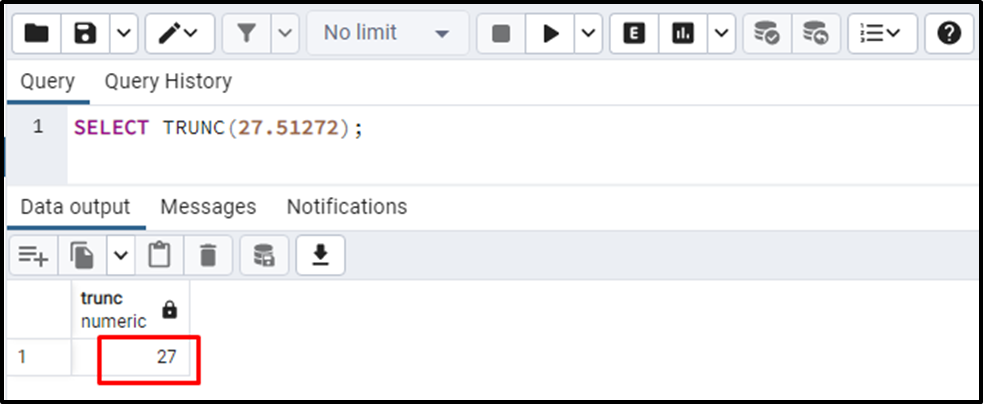The output shows that the TRUNC() function truncates the entire fractional part from the given number.

Example 2: How Does the TRUNC() Function Work With Two Parameters?

Let’s pass two arguments to the TRUNC() function and see how it works:

`SELECT TRUNC(27.51272, 2);`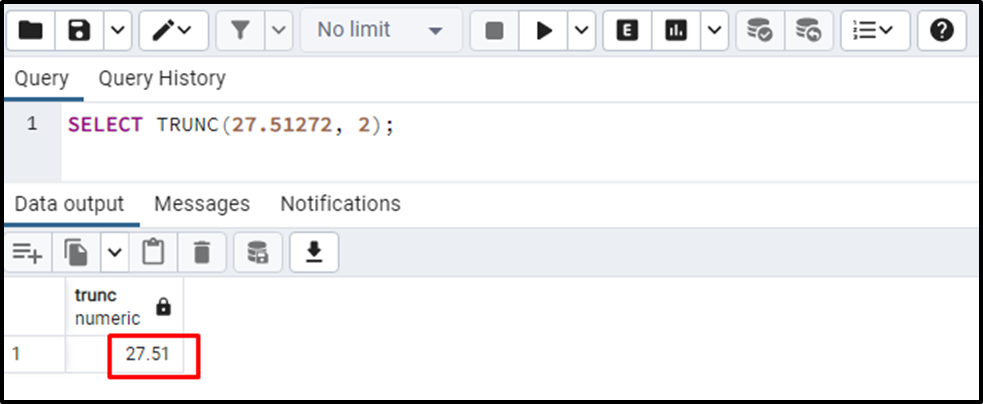The output shows that the TRUNC() function truncates the given number to two decimal places.

Example 3: How Does the TRUNC() Function Work With a Negative Parameter?

In this example, we will pass a negative value as a second parameter to see how the TRUNC() function works in such a situation:

`SELECT TRUNC(2751.272, -2);`

In the above snippet, the second argument is “-2”, so the TRUNC() function will truncate the digits to the left of the fractional/decimal point: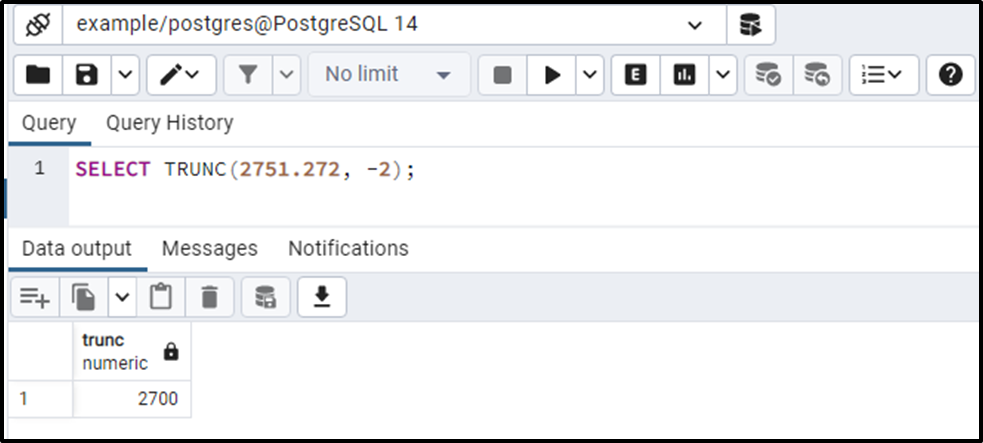This is how the TRUNC() function works with negative parameters.

Example 4: How to Use the TRUNC() Function on Table’s Data?

Firstly, we will create a sample table named “emp_bil” with three columns: emp_id, emp_name, and emp_bio:

```CREATE TABLE emp_bio(
emp_id INT,
emp_name TEXT,
emp_sal NUMERIC
);```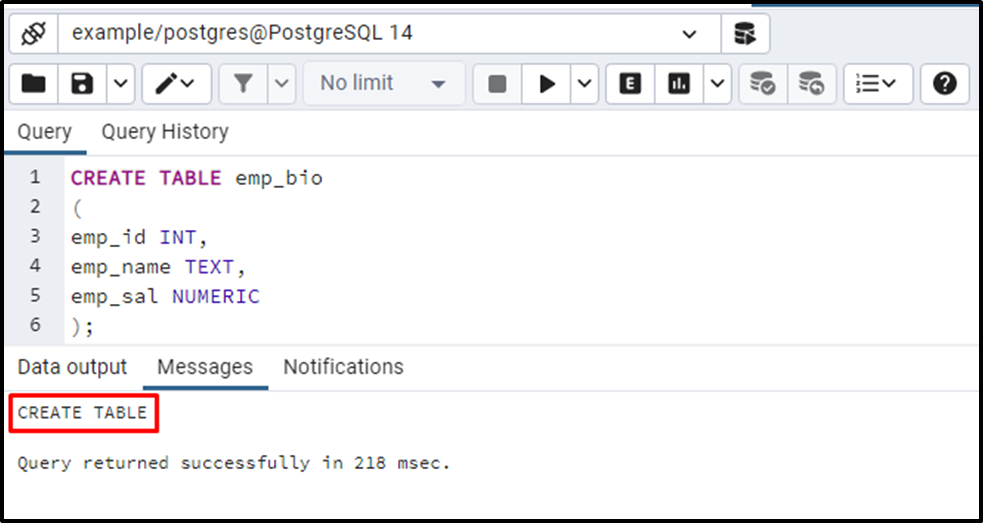The “emp_bio” is successfully created. Now, we will insert some records/data in the newly created table:

```INSERT INTO emp_bio(emp_id, emp_name, emp_sal)
VALUES
(1, 'Joe', 50500.7895),
(2, 'Kane', 40800.1234),
(3, 'Smith', 30700.5678);```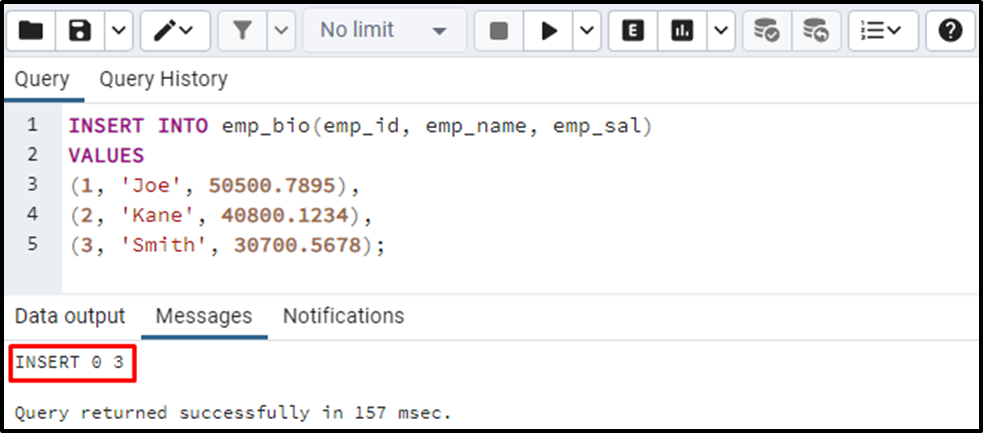Now let’s utilize the TRUNC() function on the emp_sal column to truncate the fractional part:

```SELECT TRUNC(emp_sal)
FROM emp_bio;```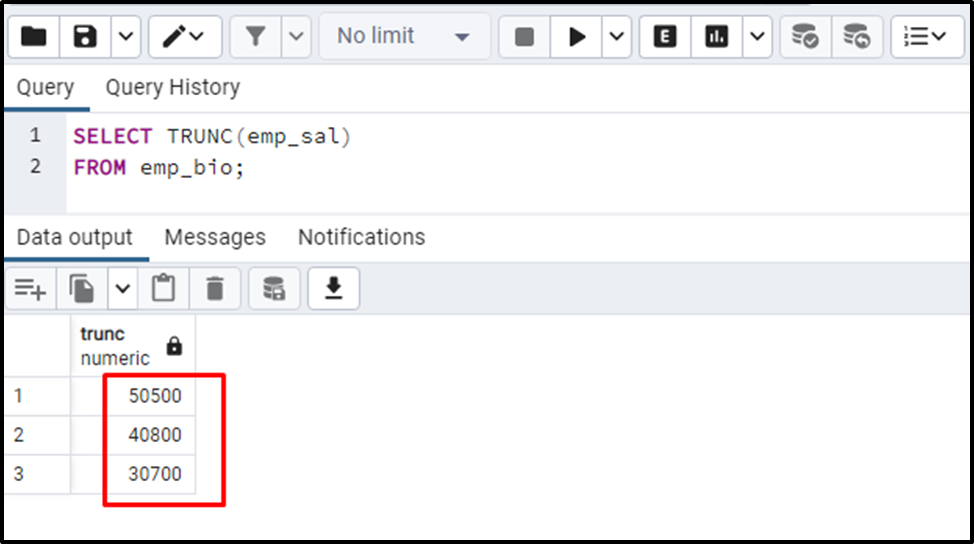The output proves that the TRUNC() function successfully truncated the fractional part from the column’s values.

Conclusion

In PostgreSQL, TRUN() function is one of the math functions that accept a numeric value and truncates its entire fractional part or up to specific decimal places. It accepts two values as arguments, a value to be truncated and a number that determines the number of decimal places. The second argument can be omitted/skipped. In such a case, the TRUNC() function will truncate the whole fractional part from the given number. This blog post presented a detailed guide on TRUNC() function via practical examples.